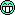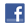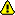Get 1 free lesson per week // Add a new lessonNew account
4 million accounts created!

• Home
• Print
• Guestbook
• Report a bug

•Get a free English lesson every week!

Partners:
- Our other sites

Learn English > English lessons and exercises > English test #2809: Why not with your fingers ?> Other English exercises on the same topic: Numbers [Change theme] > Similar tests: - Cardinal and ordinal numbers - Fractions and percentages - ESL-Numbers from 1 to 10 - Geometric shapes - Vocabulary: mathematics - Geometry-Vocabulary - Idioms: numbers - Numbers and dates... (Level 1) > Double-click on words you don't understand

# Why not with your fingers ?Add the number of fingers and  write the sum in lettersTwitter Share
English exercise "Why not with your fingers ?" created by bridg with The test builder. [More lessons & exercises from bridg]Please log in to save your progress.

1.+++=

2.=

3.+++=

4.+=

5.+++=

6.+=

7.++=

8.++=

9.=

10.+++=

11.++=

12.+++=

13.+=

14.++=

15.++=

16.+=

17.++=

18.+=

19.++=

20.+=

End of the free exercise to learn English: Why not with your fingers ?
A free English exercise to learn English.
Other English exercises on the same topic : Numbers | All our lessons and exercises